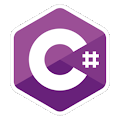Programming Course in C# ¡Free!

# Double, reference parameters

Proposed exercise

Create a function named "Double" to calculate the double of an integer number, and modify the data passed as an argument. It must be a "void" function and you must use "refererence parameters". For example.

x = 5;
Double(ref x);
Console.Write(x);

would display 10

Output

Solution

```using System;
public class F_DoubleRef
{
public static void Double(ref int n)
{
n = n + n;
}

public static void Main()
{
int x = 2;

Double( ref x );
Console.WriteLine( x );
}
}
```